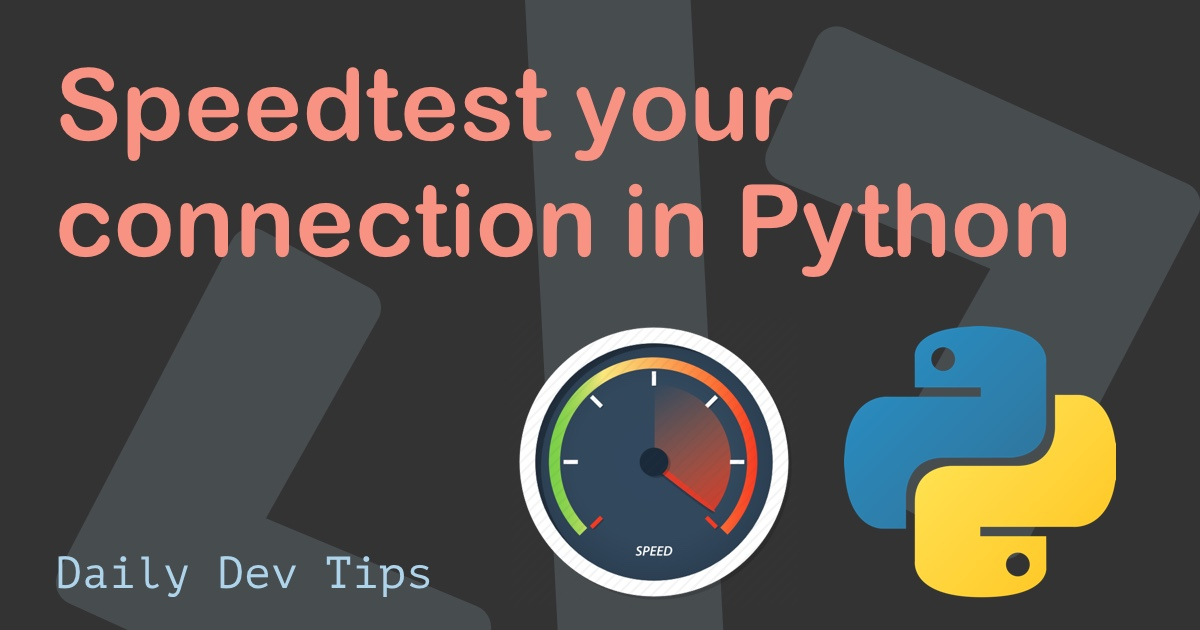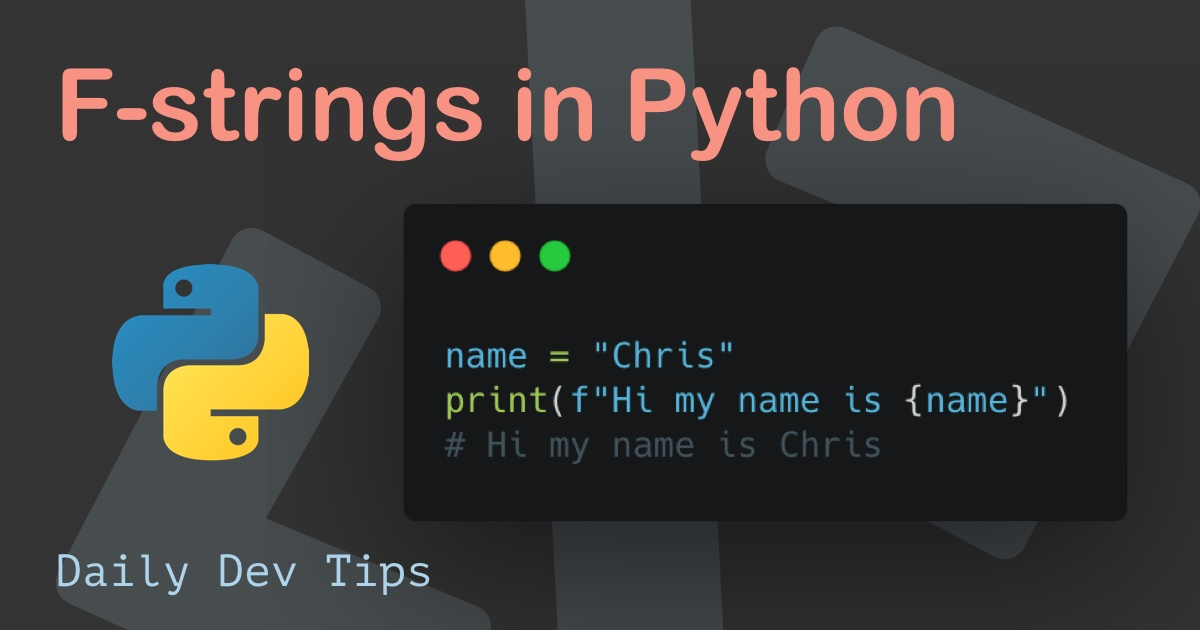Subscribe

# Python loops explained

•••• 3 devs liked it

✍️

The for and while loop in Python

24 May, 2021 · 2 min read

Besides the Python data types I've walked through just the other day, loops are an essential part of programming.

Today we'll be looking at loops in Python, and there are two types I'll explain in this article.

• For loop
• While loopLet me first show you how a basic for loop in Python looks:

``````for x in y:
# Do something``````

Based on this example, you can already see it translates to: For each element X inside of statement Y, Evaluate a code block.

Let's say we have a list with animals and would like to print each animal.

``````animals = ["cat", "dog", "penguin", "tiger"]
for animal in animals:
print(animal)``````

This will result in:

``````cat
dog
penguin
tiger``````

We can also use the range to loop x amount of times. Let's say we want to make a loop go four times.

``````for item in range(4):
print("Happy Birthday")``````

Wil print out:

``````Happy Birthday
Happy Birthday
Happy Birthday
Happy Birthday``````

Besides the for loop, there is also the option to loop while a certain condition is met.

The basics for a while loop are like this:

``````while x == True:
# Do something``````

With that, we say while X is true, you must keep executing this code block. If we actually used the code above, we would, however, build an infinite loop.

So let's make a basic while loop and break it after the first run, so it only executes once

``````foo = True
while foo == True:
print("Foo is true")
foo = False

print("Foo is false now!")``````

And this code block will return the following:

``````Foo is true
Foo is false now!``````

You've seen the range option, but we could also use a while loop for that.

``````number = 2

while number < 5:
print("Still smaller")
number = number + 1``````

This gives us:

``````Still smaller
Still smaller
Still smaller``````

And there, you have the basics of two versions to loop code blocks in Python.

Tweet this tip
•••• 3 devs liked it

### Speedtest your connection in Python

21 Jun, 2021 · 3 min read### F-strings in Python

7 Jun, 2021 · 2 min readJoin 1509 devs and subscribe to my newsletter

• 827 articles written
• 1509 devs subscribed
• 493903 words written